﻿ 地方政府支出有偏性、企业融资约束与经济结构失衡
 财经研究2016, Vol. 42Issue (10): 155-1780

#### 文章信息财经研究2016年42卷第10期

Wang Wenfu, Wang Dexin, Yue Chaoyun.

The Bias of Local Government Expenditures, Corporate Financing Constraints and the Imbalance in Economic Structure

Journal of Finance and Economics, 2016, 42(10): 155-178.

### 文章历史《财经研究》
2016第42卷第10期

1.西南财经大学 财政税务学院，四川 成都 611130;
2.西南大学 政治与公共管理学院，重庆 400700;
3.云南省社会科学院 经济研究所，云南 昆明 650034

The Bias of Local Government Expenditures, Corporate Financing Constraints and the Imbalance in Economic Structure
Wang Wenfu1, Wang Dexin1,2, Yue Chaoyun3
1.School of Public Finance and Taxation, Southwestern University of Finance and Economics, Chengdu 611130, China;
2.School of Political Science and Public Administration, Southwest University, Chongqing 400700, China;
3.Institute of Economics, Yunnan Academy of Social Sciences, Kunming 650034, China
Abstract: Based on the structure reality problems exercising current economic growth in China from a perspective of local governments, this paper uses the provincial annual data from 1993 to 2012, draws lessons from Bernanke et al. (1999) and Fernández-Villaverde (2010) and constructs a DSGE model to make an empirical study of the internal logic relationship between the imbalance in economic structure and the increase in government investment resulting from the bias of local government expenditures. It comes to the results as follows: firstly, along with economic growth, the proportions of social investment in East China, Central China and West China constantly rise, but the proportions of household consumption and labor income show the downward trends; and the performance of the imbalance in economic structure coexists with the increase in government investment resulting from the bias of local government expenditures; secondly, a simulation comparison between big enterprise model, small and medium sized enterprise model, and sticky price model shows that the aim of economic development advanced by local governments promotes the bias of local government expenditures, leading to the increase in government investment by a big margin; owing to financing constraints, the investment of small and medium sized enterprises experiences no great increase, but the investment of large enterprises experiences a big rise, thereby intensifying the imbalance in local economic structure; and in this transmission mechanism, positive externalities of government investment, corporate financing constraints and financial friction play the important role.
Key words: local government    bias of government expenditures    corporate financing constraint    imbalance in economic structure

(一) 数据说明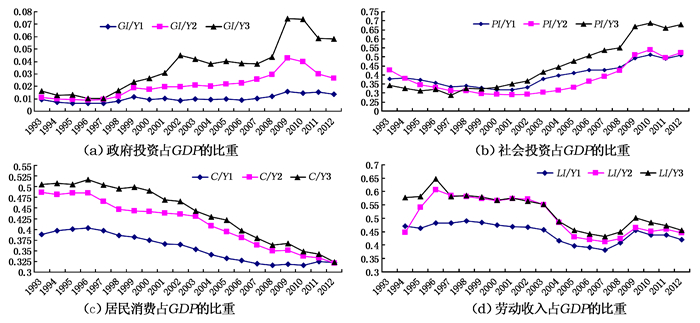图 1 东、中、西部的地方政府投资占GDP的比重与经济结构失衡的变化趋势(1993-2012年) 注：GI/Y1、GI/Y2和GI/Y3分别表示东、中、西部政府投资占总产量的比重；PI/Y1、 PI/Y2和PI/Y3分别表示东、中、西部社会投资占总产量的比重；C/Y1、 C/Y2和C/Y3分别表示东、中、西部居民消费占总产量的比重；LI/Y1、 LI/Y2和LI/Y3分别表示东、中、西部劳动收入占总收入的比重。
(二) 特征事实

(一) 基于政府与体制方面的解释

(二) 基于人口结构与经济结构的解释

(三) 基于经济增长角度的解释

(四) 基于技术进步与非完全竞争因素的解释

(五) 基于收入分配和边际消费倾向因素的解释

(六) 不确定支出预期和个体理性角度的解释

(二) 模型的建立和结构

1.厂商的优化行为。

(1)最终品厂商的最大化问题。假设经济中有一个生产最终品厂商，其投入品或生产要素来自中间品厂商生产出的产品。设该厂商的生产函数为：

 ${{y}_{t}}={{\left( \int_{0}^{1}{y_{it}^{\frac{\varepsilon -1}{\varepsilon }}di} \right)}^{\frac{\varepsilon }{\varepsilon -1}}}$ (1)

(2)中间品厂商的优化行为。假设存在大量中间品厂商，他们连续地构成了一个集合(continuum)，按照Glomm和Ravikumar(1994)的做法，假设代表性厂商的生产函数满足柯布-道格拉斯(Cobb-Douglas)形式。

 ${{y}_{it}}={{e}^{{{z}_{t}}}}k_{gt}^{\varphi }k_{it-1}^{\alpha }l_{it}^{1-\alpha }$ (2)

 $\underset{{{p}_{it}}}{\mathop{\text{Max}}}\,E\sum\limits_{\tau =0}^{\infty }{{{\left( \beta \theta \right)}^{\tau }}}\frac{{{\lambda }_{t+\tau }}}{{{\lambda }_{t}}}\left\{ \left\{ \prod\limits_{s=1}^{\tau }{\frac{\prod\nolimits_{t+s-1}^{\chi }{{}}}{\prod\nolimits_{t+s}{{}}}\frac{{{p}_{it}}}{{{p}_{t}}}-m{{c}_{t+\tau }}} \right\}{{y}_{it}} \right\}$ (3)

 ${{y}_{it+\tau }}={{\left\{ \prod\limits_{s=1}^{\tau }{\frac{\prod\nolimits_{t+s-1}^{\chi }{{}}}{\prod\nolimits_{t+s}{{}}}\frac{{{p}_{it}}}{{{p}_{t}}}} \right\}}^{-\varepsilon }}{{y}_{t+\tau }}$ (4)

(3)资本品生产者的行为。假设资本品市场是完全竞争型的，其中代表性资本品生产者购买安装资本xt，同时使用最终产品作为投资量，生产出下期的安装成本。

 ${{x}_{t+1}}={{x}_{t}}+\left( 1-S\left[ \frac{{{i}_{t}}}{{{i}_{t-1}}} \right] \right){{i}_{t}}$ (5)

 ${{k}_{t+1}}={{k}_{t}}+\left( 1-S\left[ \frac{{{i}_{t}}}{{{i}_{t-1}}} \right] \right){{i}_{t}}$ (6)

 ${{k}_{gt}}=\left( 1-\delta \right){{k}_{gt-1}}+{{I}_{gt}}$ (7)

(4)债务合同企业和金融中介。在此假设存在代表性具有债务合同的企业，以下简称为债务合同企业。其主要目的是从资本品生产者那儿购买资本品，然后把资本品租赁给中间品厂商，从而实现其利润的最大化。同时，假设代表性具有债务合同的企业使用每期末的真实财富nt和名义贷款额bt，去购买新安装成本：

 ${{q}_{t}}{{k}_{t}}={{n}_{t}}+\frac{{{b}_{t}}}{{{p}_{t}}}$ (8)

 $R_{t+1}^{k}=\left( \frac{{{p}_{t+1}}}{{{p}_{t}}} \right)\left( \frac{{{r}_{t+1}}+{{q}_{t+1}}\left( 1-\delta \right)}{{{q}_{t}}} \right)$ (9)

 $R_{t+1}^{l}{{b}_{t}}={{{\bar{\omega }}}_{t+1}}R_{t+1}^{k}{{p}_{t}}{{q}_{t}}{{k}_{t}}$ (10)

 \begin{align} & \left[ 1-F\left( {{{\bar{\omega }}}_{t+1}},{{\sigma }_{\omega ,t+1}} \right) \right]R_{t+1}^{l}{{b}_{t}}+\left( 1-\mu \right) \\ & \int_{0}^{{{{\bar{\omega }}}_{t+1}}}{\omega dF\left( {{\omega }_{t+1}},{{\sigma }_{\omega ,t+1}} \right)}R_{t+1}^{K}{{p}_{t}}{{q}_{t}}{{k}_{t}}={{s}_{t}}{{R}_{t}}{{b}_{t}} \\ \end{align} (11)

 $\underset{\rho t,\bar{\omega }t+1}{\mathop{\text{Max}}}\,{{E}_{t}}\left\{ \frac{R_{t+1}^{k}}{{{R}_{t}}}\left[ 1-\Gamma \left( {{{\bar{\omega }}}_{t+1}},{{\sigma }_{\omega ,t+1}} \right) \right]\left( 1+{{\rho }_{t}} \right) \right\}{{R}_{t}}{{n}_{t}}$ (12)
 $\text{s}\text{.t}\text{.}\frac{R_{t+1}^{k}}{{{s}_{t}}{{R}_{t}}}\left( \Gamma \left( {{{\bar{\omega }}}_{t+1}},{{\sigma }_{\omega ,t+1}} \right)-\mu G\left( {{{\bar{\omega }}}_{t+1}},{{\sigma }_{\omega ,t+1}} \right) \right)\left( 1+{{\rho }_{t}} \right)={{\rho }_{t}}$ (13)

 \begin{align} & {{p}_{t}}{{n}_{t}}=R_{t}^{k}{{p}_{t-1}}{{q}_{t-1}}{{k}_{t-1}}-{{s}_{t-1}}{{R}_{t-1}}{{b}_{t-1}}- \\ & \mu \int_{0}^{{{{\bar{\omega }}}_{t+1}}}{\omega dF\left( {{{\bar{\omega }}}_{t+1}},{{\sigma }_{\omega ,t+1}} \right)R_{t}^{k}{{p}_{t-1}}{{q}_{t-1}}{{k}_{t-1}}+{{p}_{t}}{{\omega }^{e}}} \\ \end{align} (14)

2.家庭的优化行为。代表性家庭的目标是最大化一生的效用。

 ${{E}_{0}}\sum\limits_{t=0}^{\infty }{{{\beta }^{t}}}\left[ \log \left( {{c}_{t}}-h{{c}_{t-1}} \right)-\psi \frac{l_{t}^{1+\vartheta }}{1-\vartheta }+\upsilon \log \left( \frac{{{m}_{t-1}}}{{{p}_{t}}} \right) \right]$ (15)

 \begin{align} & \left( 1+{{\tau }_{c,t}} \right){{c}_{t}}+\frac{{{a}_{t}}}{{{p}_{t}}}+\frac{{{d}_{t}}}{{{p}_{t}}}+\frac{{{m}_{t}}}{{{p}_{t}}}= \\ & \left( 1-{{\tau }_{l,t}} \right){{\omega }_{t}}{{l}_{t}}+\left[ 1+\left( 1-{{\tau }_{R,t}} \right)\left( {{\tau }_{R,t}}-1 \right) \right]\frac{{{a}_{t-1}}}{{{p}_{t}}} \\ & +R{{d}_{t-1}}\frac{{{d}_{t-1}}}{{{p}_{t}}}+\frac{{{m}_{t-1}}}{{{p}_{t}}}+{{T}_{t}}+{{F}_{t}}+tr{{e}_{t}} \\ \end{align} (16)

3.政府部门的行为。

(1)货币当局。假设货币当局按照以下泰勒规则设定利率政策

 $\frac{{{R}_{t}}}{R}={{\left( \frac{{{R}_{t-1}}}{R} \right)}^{{{\gamma }_{R}}}}{{\left[ {{\left( \frac{{{\Pi }_{t}}}{\Pi } \right)}^{{{\gamma }_{\Pi }}}}{{\left( \frac{{{y}_{t}}}{y} \right)}^{{{\gamma }_{y}}}} \right]}^{1-{{\gamma }_{R}}}}$ (17)

(2)财政部门。假设财政预算的跨期约束为：

 $\frac{{{d}_{t}}}{{{p}_{t}}}={{g}_{t}}+R{{d}_{t-1}}\frac{{{d}_{t-1}}}{{{p}_{t}}}-ta{{x}_{t}}$ (18)
 ${{g}_{t}}={{c}_{gt}}+{{i}_{gt}}$ (19)

 $ta{{x}_{t}}={{\tau }_{c,t}}{{c}_{t}}+{{\tau }_{l,t}}{{\omega }_{t}}{{l}_{t}}+\left( 1-{{\tau }_{R,t}} \right)\left( {{R}_{t-1}}-1 \right)\frac{{{a}_{t-1}}}{{{p}_{t}}}$ (20)

 ${{{\hat{i}}}_{gt}}={{\gamma }_{h}}{{{\hat{i}}}_{gt-1}}+{{\varepsilon }_{g,t}}$ (21)

4.市场均衡。产品市场的均衡条件为：

 ${{y}_{t}}={{c}_{t}}+{{i}_{t}}+{{g}_{t}}+\mu G\left( {{{\bar{\omega }}}_{t+1}},{{\sigma }_{\omega ,t+1}} \right)\left( {{r}_{t}}+{{q}_{t}}\left( 1-\delta \right) \right){{k}_{t-1}}$ (22)

 ${{l}_{t}}=\int_{0}^{1}{{{l}_{t}}\left( j \right)dj}$ (23)

 ${{k}_{t}}=\int_{0}^{1}{{{k}_{t}}\left( j \right)dj}$ (24)

 ${{y}_{it}}={{e}^{{{z}_{t}}}}k_{gt}^{\varphi }k_{ik-1}^{\alpha }l_{ik}^{1-\alpha }$ (25)
(三) 对数线性化的经济系统

 \left\{ \begin{align} & {{c}_{t}},{{\lambda }_{t}},{{l}_{t}},{{r}_{t}},{{\omega }_{t}},m{{c}_{t}},{{\Pi }_{t}},\Pi _{t}^{*},{{{\bar{\omega }}}_{t}},{{b}_{t}}/{{p}_{t}},{{n}_{t}},{{q}_{t}},{{k}_{t}},{{d}_{t}},{{R}_{t}}, \\ & R{{d}_{t}},R_{t}^{k},{{y}_{t}},{{v}_{t}},{{i}_{t}},{{z}_{t}},{{g}_{t}},{{c}_{gt}},{{i}_{gt}},ta{{x}_{t}},{{{\hat{\tau }}}_{t}} \\ \end{align} \right\}

(四) 模型的参数校准(见表 1表 2表 3)

 α δ εp θ η R s γ χ σω u β 0.56 0.025 4.60 0.75 1 1.025 1.99 1.73 0.60 0.50 0.10 0.99 h τ φπ φπ ρG σG ρT π γR d dg b/k 0.58 0.16 1.8 0.25 0.57 0.038 0.23 1.02 0.95 0 0.18 2/3

 α δ εp θ η R s γ χ σω u β 0.33 0.025 4.60 0.75 1 1.025 5.99 1.73 0.60 0.50 0.50 0.99 h τ φπ φπ ρG σG ρT π γR d dg b/k 0.58 0.16 1.8 0.25 0.57 0.038 0.23 1.02 0.95 0 0.18 1/3

 α δ εp θ η R χ u β h 0.50 0.025 4.60 0.75 1 1.025 0.60 0.30 0.99 0.58 τ φπ φπ ρG σG ρT π γR d dg 0.16 1.8 0.25 0.57 0.038 0.23 1.02 0.95 0 0.18

1.厂商生产参数。一是关于资本的产出弹性α。国内学者对资本产出弹性的估计在0.5左右，如王小鲁和樊纲(2000)的估计为0.5，而张军(2002)的估计值为0.499。但是国内没有对大企业和中小企业的资本产出弹性进行估计，鉴于大企业和中小企业在资本和劳动上的密集度差异，前者的资本产出弹性大于0.5，后者的资本产出弹性小于0.5。于是我们把大企业和中小企业的资本产出弹性分别取为0.56和0.33。二是关于资本折旧率的确定。按照陈昆亭和龚六堂(2005)的做法，本文选取的年折旧率为0.10，这意味着固定资产的平均使用年限为10年，取其季度平均值，表示季度折旧率δ=0.025。三是关于最终品对中间品价格需求弹性εp的确定。陈昆亭等(2004)取其为10，李春吉和孟晓宏(2006)由模型模拟所获值为εp=3.7064，而Zhang(2009)的估计值为εp=4.61，本文取εp=4.6。四是关于每期保持价格不变的中间厂商占社会比重。陈昆亭和龚六堂(2006)取θ=0.6，而Zhang(2009)基于GMM实证数据得到θ=0.84，而梅冬州、龚六堂(2011)取θ=0.75，这意味着所有商品价格每年调整一次，本文也取此值。五是关于托宾Q的投资弹性的确定。本文按照王君斌等(2011)的做法取值为η=1。六是关于稳态处名义毛利率R的确定。本文取R≡r+1=1.025。价格指数化加成参数校准为χ=0.6。需要说明的是，关于资本的折旧率、最终品厂商对中间品价格需求的弹性、托宾Q的投资弹性、稳态处的名义毛利率、价格指数化加成参数，我们对大企业和中小企业的取值基本相同，因为我们在模拟时分析发现这些值的变化对本文的分析结论影响不大。

2.居民偏好参数。关于居民的主观贴现率。我们选用1992年第1季度至2013年第3季度的CPI季度数据，发现CPI季度增长率为1.09%，故在此取家庭主观效用的贴现率为β=0.99。关于消费习惯参数，国内DSGE框架中引入消费习惯这一概念的不多，我们参照王君斌等(2011)的做法，取值h=0.583。关于劳动供给对工资的弹性，在此按照梅冬州和龚六堂(2011)做法，取值为0.30。

3.政府政策参数。根据中经专网统计数据库，可得到1992年第1季度至2013年第3季度相关财政政策的数据，取其平均税率的均值，把它作为稳态时的收入税率τ=0.15。对货币利率简单泰勒规则系数的处理，石柱鲜等(2009)的研究表明，在泰勒利率规则方程中，通货膨胀缺口系数φπ=1.766，产出通货膨胀缺口系数φπ=0.2533。本文的模拟就以他们的参数为基础。关于政府支出和收入税率序列的处理，对波动部分进行无截距的一阶自回归可得：政府支出冲击自相关系数ρG=0.57，标准差为σG=3.76%；收入税率冲击自相关系数ρT=0.227，标准差为σT=3.07%。由李春吉和孟晓宏(2006)的年度稳态通货膨胀率为1.08，于是设定季度稳态通货膨胀率π=1.02。

4.金融加速器相关参数。关于金融合同隐含的稳态企业资产与净资产比例K/N，大企业的这一参数值比中小企业高，我们对大企业值取为2，对中小企业取值为0.5。而关于债务合同企业从金融中介融资的稳态值，大企业对应的值应该较低，取值为s=1.99；而中小企业对应的值较高，取值为s=5.99。此外，中国金融中介对大企业的监管较容易，而对中小企业的监管则较难。于是企业一旦破产，金融中介从大企业拿到剩余资产的份额就比中小企业多，假设大企业破产后交给金融中介的剩余资产份额为(1-u)=0.9或u=0.10，假设中小企业破产后交给金融中介的剩余资产份额为(1-u)=0.50或u=0.50。同时，参照(Fernández-Villaverde，2010)的取值，我们取与金融合同有关的参数校准值分别为γ=1.73和σw=0.50。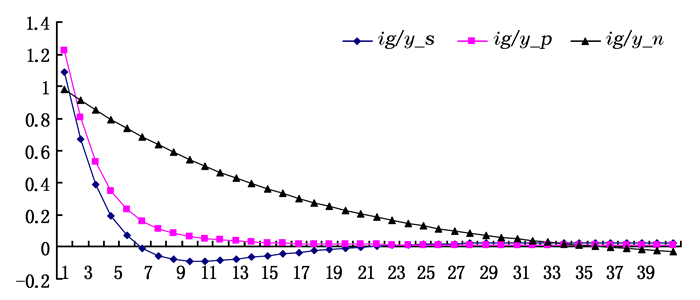图 2 政府投资占总产量的比重对政府投资冲击的动态响应 注：ig/y_s、ig/y_p和ig/y_n分别表示在大企业模型、中小企业模型和价格粘性模型中政府投资占总产量的比重对政府投资冲击的动态响应。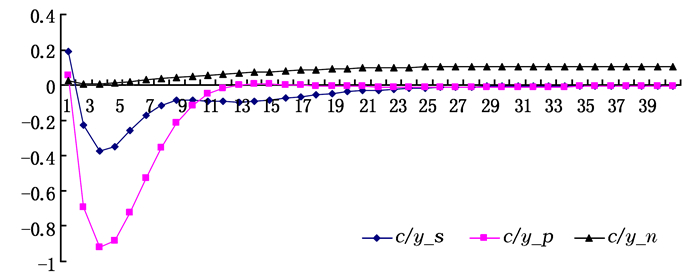图 3 居民消费占总收入的比重对政府投资冲击的动态响应 注：c/y_s、c/y_p和c/y_n分别表示在大企业模型、中小企业模型和价格粘性模型中居民消费占总收入的比重对政府投资冲击的动态响应。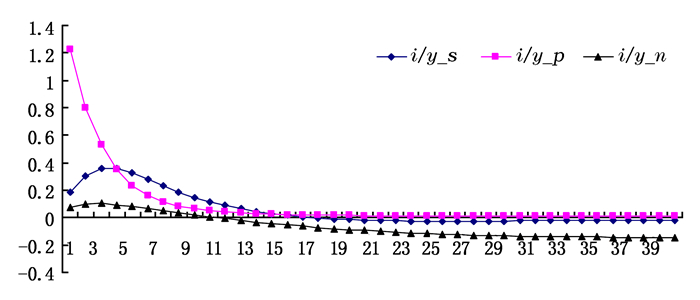图 4 企业投资占总产量的比重对政府投资冲击的动态响应 注：i/y_s、i/y_p和i/y_n分别表示在大企业模型、中小企业模型和粘性价格模型中企业投资占总产量的比重对政府投资冲击的动态响应。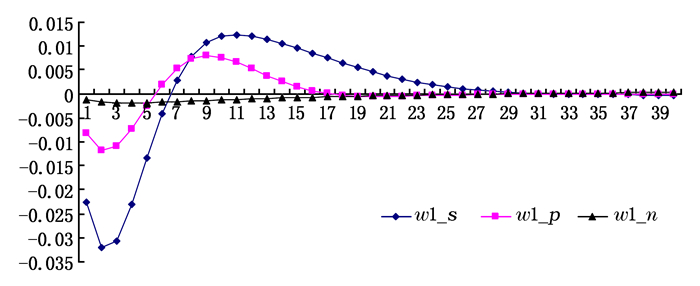图 5 劳动收入占总收入的比重对政府投资冲击的动态响应 注：w1_s、w1_p和w1_n分别表示在大企业模型、中小企业模型和价格粘性模型中劳动收入占总收入的比重对政府投资冲击的动态响应。

(二) 传导机制的分析

  艾春荣, 汪伟. 习惯偏好下的中国居民消费的过度敏感性——基于1995-2005年省际动态面板数据的分析[J]. 数量经济技术经济研究 , 2008 (11) : 98–114.  安苑, 王珺. 财政行为波动影响产业结构升级了吗?[J]. 管理世界 , 2012 (9) : 19–35.  陈昆亭, 龚六堂, 邹恒甫. 什么造成了经济增长的波动, 供给还是需求——中国经济的RBC分析[J]. 世界经济 , 2004 (4) : 3–11.  陈昆亭, 龚六堂. 粘滞价格模型以及对中国经济的数值模拟——对基本RBC模型的改进[J]. 数量经济技术经济研究 , 2006 (8) : 106–117.  陈利平. 高储蓄率并非需求不足的根源[J]. 经济研究 , 2001 (3) : 46–50.  陈利平. 高增长导致高储蓄:一个基于消费攀比的解释[J]. 世界经济 , 2005 (11) : 3–9.  程令国, 张晔. 早年的饥荒经历影响了人们的储蓄行为吗?——对我国居民高储蓄率的一个新解释[J]. 经济研究 , 2011 (8) : 119–132.  崔光灿. 资产价格、金融加速器与经济稳定[J]. 世界经济 , 2006 (11) : 59–69.  方福前. 中国居民消费需求不足原因研究——基于中国城乡分省数据[J]. 中国社会科学 , 2009 (2) : 68–82.  方红生, 张军. 中国地方政府竞争、预算软约束与扩张偏向的财政行为[J]. 经济研究 , 2009 (12) : 4–16.  傅勇, 张晏. 中国式分权与财政支出结构偏向:为增长而竞争的代价[J]. 管理世界 , 2007 (3) : 4–22.  杭斌. 城镇居民的平均消费倾向为何持续下降——基于消费习惯形成的实证分析[J]. 数量经济技术经济研究 , 2010 (6) : 126–138.  何刚, 陈文静. 公共资本和私人资本的生产效率及其区域差异——基于分位数回归模型的研究[J]. 数量经济技术经济研究 , 2008 (9) : 42–51.  黄赜琳. 中国经济周期特征与财政政策效应——一个基于三部门RBC模型的实证分析[J]. 经济研究 , 2005 (6) : 27–39.  黄先海, 徐圣. 中国劳动收入比重下降成因分析——基于劳动节约型技术进步的视角[J]. 经济研究 , 2009 (7) : 34–44.  胡永刚, 郭新强. 内生增长、政府生产性支出与中国居民消费[J]. 经济研究 , 2012 (9) : 57–70.  李承政, 邱俊杰. 中国农村人口结构与居民消费研究[J]. 人口与经济 , 2012 (1) : 49–56.  李春吉, 孟晓宏. 中国经济波动——基于新凯恩斯主义垄断竞争模型分析[J]. 经济研究 , 2006 (10) : 72–82.  李稻葵, 何梦杰, 刘霖林. 我国现阶段初次分配中劳动收入下降分析[J]. 经济理论与经济管理 , 2010 (2) : 13–19.  李扬, 殷剑峰. 中国高储蓄率问题探究——1992-2003年中国资金流量表的分析[J]. 经济研究 , 2007 (6) : 14–26.  李扬, 殷剑峰, 陈洪波. 中国:高储蓄、高投资和高增长研究[J]. 财贸经济 , 2007 (1) : 26–33.  李永友. 需求结构失衡的财政因素:一个分析框架[J]. 财贸经济 , 2010 (11) : 63–70.  刘斌. 我国DSGE模型的开发及在货币政策分析中的应用[J]. 金融研究 , 2008 (10) : 1–21.  刘生龙. 基础设施的外部性在中国的检验:1988—2007[J]. 经济研究 , 2010 (3) : 4–15.  柳庆刚, 姚洋. 地方政府竞争和结构失衡[J]. 世界经济 , 2012 (12) : 3–22.  柳欣, 赵雷, 吕元祥. 我国经济增长中的需求结构失衡探源——基于存量-流量均衡的分析视角[J]. 经济学动态 , 2012 (7) : 57–63.  娄峰, 李雪松. 中国城镇居民消费需求的动态实证分析[J]. 中国社会科学 , 2009 (3) : 109–115.  罗长远. 卡尔多"特征事实"再思考:对劳动收入占比的分析[J]. 世界经济 , 2008 (11) : 86–96.  罗长远, 张军. 经济发展中的劳动收入占比:基于中国产业数据的实证研究[J]. 中国社会科学 , 2009a (4) : 65–79.  罗长远, 张军. 劳动收入占比下降的经济学解释——基于中国省级面板数据的分析[J]. 管理世界 , 2009b (5) : 25–35.  罗楚亮. 经济转轨、不确定性与城镇居民消费行为[J]. 经济研究 , 2004 (4) : 100–106.  吕炜. 体制性约束、经济失衡与财政政策——解析1998年以来的中国转轨经济[J]. 中国社会科学 , 2004 (2) : 4–17.  梅冬州, 龚六堂. 新兴市场经济国家的汇率制度选择[J]. 经济研究 , 2011 (11) : 73–88.  渠慎宁, 吴利学, 夏杰长. 中国居民消费价格波动:价格粘性、定价模式及其政策含义[J]. 经济研究 , 2012 (11) : 88–102.  沈坤荣, 付文林. 中国的财政分权制度与地区经济增长[J]. 管理世界 , 2005 (1) : 31–39.  石奇, 孔群喜. 动态效率、生产性公共支出与结构效应[J]. 经济研究 , 2012 (1) : 92–104.  石柱鲜, 孙皓, 邓创. Taylor规则在我国货币政策中的实证检验——基于时变隐性通货膨胀目标的新证据[J]. 当代财经 , 2009 (12) : 43–48.  孙迎联. 居民消费需求影响因素分析[J]. 财经科学 , 2011 (3) : 76–82.  邰秀军, 李树茁, 李聪, 等. 中国农户谨慎性消费策略的形成机制[J]. 管理世界 , 2009 (7) : 85–92.  王弟海, 龚六堂. 增长经济中的消费和储蓄——兼论中国高储蓄率的原因[J]. 金融研究 , 2007 (12) : 1–16.  王君斌, 王文甫. 非完全竞争市场、技术冲击和中国劳动就业——动态新凯恩斯主义视角[J]. 管理世界 , 2010 (1) : 23–43.  王君斌, 郭新强, 蔡建波. 扩张性货币政策下的产出超调、消费抑制和通货膨胀惯性[J]. 管理世界 , 2011 (3) : 7–21.  王小鲁, 樊纲. 我国工业增长的可持续性[M]. 北京: 经济科学出版社, 2000 .  汪伟, 郭新强. 收入不平等与中国高储蓄率:基于目标性消费视角的理论与实证研究[J]. 管理世界 , 2011 (9) : 7–26.  伍山林. 劳动收入份额决定机制:一个微观模型[J]. 经济研究 , 2011 (9) : 55–68.  徐忠, 张雪春, 丁志杰, 等. 公共财政与中国国民收入的高储蓄倾向[J]. 中国社会科学 , 2010 (6) : 93–107.  闫新华, 杭斌. 内、外部习惯形成及居民消费结构——基于中国农村居民的实证研究[J]. 统计研究 , 2010 (5) : 32–40.  杨汝岱, 朱诗娥. 公平与效率不可兼得吗?——基于居民边际消费倾向的研究[J]. 经济研究 , 2007 (12) : 46–58.  杨胜刚, 侯坤. 中国是否存在金融加速器效应?——基于VEC模型的实证检验[J]. 经济管理 , 2011 (7) : 9–15.  袁申国, 陈平, 刘兰凤. 汇率制度、金融加速器和经济波动[J]. 经济研究 , 2011 (1) : 57–70.  张军. 资本形成、工业化与经济增长:中国的转轨特征[J]. 经济研究 , 2002 (6) : 3–13.  张军, 高远, 傅勇, 等. 中国为什么拥有了良好的基础设施?[J]. 经济研究 , 2007 (3) : 4–19.  张五常. 中国的经济制度[M]. 北京: 中信出版社, 2009 .  赵振全, 于震, 刘淼. 金融加速器效应在中国存在吗?[J]. 经济研究 , 2007 (6) : 27–38.  周黎安. 转型中的地方政府:官员激励与治理[M]. 上海: 格致出版社, 上海人民出版社, 2008 .  周业安, 章泉. 财政分权、经济增长和波动[J]. 管理世界 , 2008 (3) : 6–15.  朱微亮, 刘海龙, 史青青. 基于调整成本的产出——资本资产定价模型研究[J]. 管理工程学报 , 2009 (4) : 121–138.  Baxter M, King R. Fiscal policy in general equilibrium[J]. American Economic Review , 1993, 83 (3) : 315–34.  Bernanke B, Gertler M, Gilchrist S. The financial accelerator in a quantitative business cycle framework[A]. Taylor J B, Woodford M. Handbook of macroeconomics[C]. Duth:North-Holland, 1999.  Calvo G. Staggered prices in a utility-maximizing framework[J]. Journal of Monetary Economics , 1983, 12 (3) : 383–398. DOI:10.1016/0304-3932(83)90060-0  Chamon M, Prasad E. Why are saving rates of urban households in China rising?[J]. American Economic Journal: Macroeconomics , 2008, 2 (1) : 93–130.  Christiano L, Eichenbaum M, Evans C. Nominal rigidities and the dynamic effects of a shock to monetary policy[J]. Journal of Political Economy , 2005, 113 (1) : 1–45. DOI:10.1086/426038  Dixit A, Stiglitz J. Monopolistic competition and optimum product diversity[J]. American Economic Review , 1977, 67 (3) : 297–308.  Dollar D, Wei S. Das (wasted)kapital: Firm ownership and investment efficiency in China[R]. IMF Working Paper No.07/9, 2007.  Fernández-Villaverde J. Fiscal policy in a model with financial frictions[J]. American Economic Review , 2010, 100 (2) : 35–40. DOI:10.1257/aer.100.2.35  Gali J, Lopezsalido J, Valles J. Understanding the effects of government spending on consumption[J]. Journal of the European Economic Association , 2007, 5 (1) : 227–270. DOI:10.1162/jeea.2007.5.issue-1  Glomm G, Ravikumar B. Public investment in infrastructure in a simple growth model[J]. Journal of Economic Dynamics and Control , 1994, 18 (6) : 1173–1187. DOI:10.1016/0165-1889(94)90052-3  Karabarbounis L, Neiman B. The global decline of the labor share[J]. Quarterly Journal of Economics , 2013, 129 (1) : 61–103.  Kiyotaki N, Moore J. Credit cycles[J]. Journal of Political Economy , 1997, 105 (2) : 211–248. DOI:10.1086/262072  Linnemann L. The effect of government spending on private consumption: A puzzle?[J]. Journal of Money, Credit and Banking , 2006, 38 (7) : 1715–1735. DOI:10.1353/mcb.2006.0094  Perotti R. In search of the transmission mechanism of fiscal policy[J]. NBER Macroeconomics Annual , 2007, 22 : 169–249. DOI:10.1086/ma.22.25554966  RiedelJ, JinJ, GaoJ. How China grows: Investment, finance and reform[M]. Princeton, N J: Princeton University Press, 2007 .  Smets F, Wouters R. Shocks and frictions in US business cycles: A Bayesian DSGE approach[J]. American Economic Review , 2007, 97 (3) : 586–606. DOI:10.1257/aer.97.3.586  Song Z, Storesletten K, Zilibotti F. Growing like China[J]. The American Economic Review , 2011, 101 (1) : 196–233. DOI:10.1257/aer.101.1.196  Wen Y. Saving and growth under borrowing constraints explaining the 'high saving rate' puzzle[J]. Federal Reserve Bank of St. Louis Working Paper No. 2009-045C, 2010. http://www.econ.nthu.edu.tw/ezfiles/172/1172/attach/20/pta_22912_7261125_22070.pdf  Zhang W. China's monetary policy: Quantity versus price rules[J]. Journal of Macroeconomics , 2009, 31 (3) : 473–484. DOI:10.1016/j.jmacro.2008.09.003